# 基于 Keras 实现的图书推荐系统[转]

<Github - RecommendationSystem>## 1. Embedding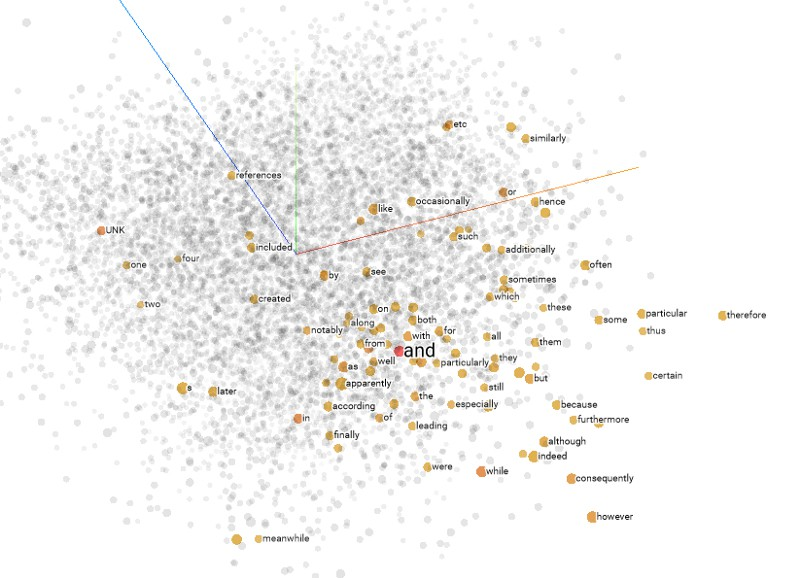Figure 2: Projector Embeddings

## 2. 获取数据

dataset = pd.read_csv('ratings.csv')
train, test = train_test_split(dataset, test_size=0.2, random_state=42)
n_users = len(dataset.user_id.unique())
n_books = len(dataset.book_id.unique())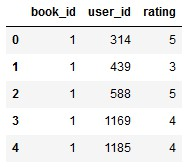## 3. 建立嵌入模型

 - 输入：包括书和用户

 - 嵌入层：书和用户的嵌入

 - 点乘：使用点乘来合并各个嵌入向量

from keras.layers import Input, Embedding, Flatten, Dot, Dense
from keras.models import Model

#  - 输入 & 嵌入层
book_input = Input(shape=, name="Book-Input")
book_embedding = Embedding(n_books, 5, name="Book-Embedding")(book_input)
book_vec = Flatten(name="Flatten-Books")(book_embedding)

user_input = Input(shape=, name="User-Input")
user_embedding = Embedding(n_users, 5, name="User-Embedding")(user_input)
user_vec = Flatten(name="Flatten-Users")(user_embedding)

#  - 点乘
prod = Dot(name="Dot-Product", axes=1)([book_vec, user_vec])
model = Model([user_input, book_input], prod)
model.compile('adam', 'mean_squared_error')

## 4. 训练模型

history = model.fit([train.user_id, train.book_id],
train.rating,
epochs=10,
verbose=1)
model.save('regression_model.h5')

## 5. 可视化嵌入

# Extract embeddings
book_em = model.get_layer('Book-Embedding')
book_em_weights = book_em.get_weights()

from sklearn.decomposition import PCA
import seaborn as sns

pca = PCA(n_components=2)
pca_result = pca.fit_transform(book_em_weights)
sns.scatterplot(x=pca_result[:,0], y=pca_result[:,1])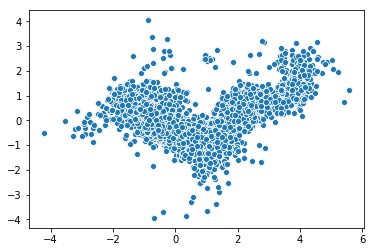Figure4: 用PCA来可视化嵌入

from sklearn.manifold import TSNE

tsne = TSNE(n_components=2, verbose=1, perplexity=40, n_iter=300)
tnse_results = tsne.fit_transform(book_em_weights)
sns.scatterplot(x=tnse_results[:,0], y=tnse_results[:,1])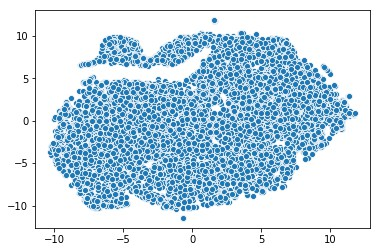Figure 5: 用t-SNE可视化嵌入

## 6. 进行推荐

# Creating dataset for making recommendations for the first user
book_data = np.array(list(set(dataset.book_id)))
user = np.array([1 for i in range(len(book_data))])
predictions = model.predict([user, book_data])
predictions = np.array([a for a in predictions])
recommended_book_ids = (-predictions).argsort()[:5]
print(recommended_book_ids)
print(predictions[recommended_book_ids])

array([4942, 7638, 8853, 9079, 9841], dtype=int64)
array([5.341809 , 5.159592 , 4.9970446, 4.9722786, 4.903894 ], dtype=float32)

books = pd.read_csv(‘books.csv’)
books.head()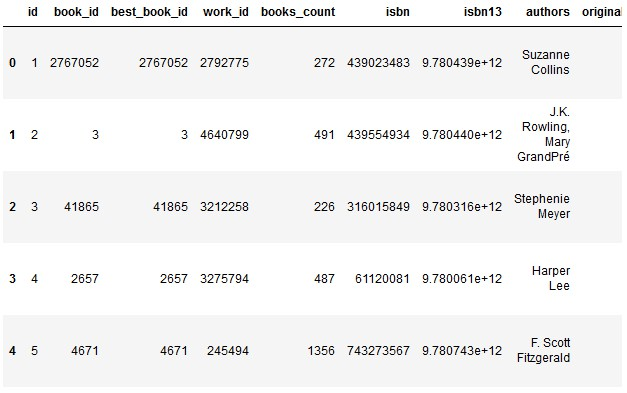print(books[books[‘id’].isin(recommended_book_ids)])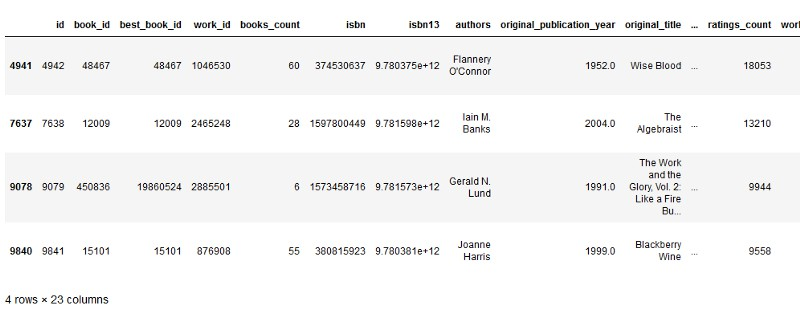## 7. 总结

• 在点乘后加入全连接层
• 训练更多轮
• 对评分列做归一化
• 等等......
Last modification：January 7th, 2019 at 08:53 pm